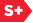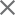### Proceedings Paper

Algorithms of the q2^r× q2^r-point 2D discrete Fourier transform
Format Member Price Non-Member Price
PDF \$17.00 \$21.00

Paper Abstract

In this paper, the concept of partitions revealing the two-dimensional discrete Fourier transform (2-D DFT) of order q2r × q2r, where r > 1 and q is a positive odd number, is described. Two methods of calculation of the 2-D DFT are analyzed. The q2r × q2r-point 2-D DFT can be calculated by the traditional column-row method with 2(q2r) 1-D DFTs, and we propose the fast algorithm which splits each 1-D DFT by the short transforms by means of the fast paired transforms. Another effective algorithm of calculation of the q2r × q2r-point 2-D DFT is based on the tensor or paired representations of the image when the image is represented as a set of 1-D signals which define the 2-D transform in the different subsets of frequency-points and they all together cover the complete set of frequencies. In this case, the splittings of the q2r × q2r-point 2-D DFT are performed by the 2-D discrete tensor or paired transforms, respectively, which lead to the calculation with a minimum number of 1-D DFTs. Examples of the transforms and computational complexity of the proposed algorithms are given.

Paper Details

Date Published: 16 March 2015
PDF: 12 pages
Proc. SPIE 9399, Image Processing: Algorithms and Systems XIII, 93990O (16 March 2015); doi: 10.1117/12.2083471
Show Author Affiliations
Artyom M. Grigoryan, The Univ. of Texas at San Antonio (United States)
Sos S. Agaian, The Univ. of Texas at San Antonio (United States)

Published in SPIE Proceedings Vol. 9399:
Image Processing: Algorithms and Systems XIII
Karen O. Egiazarian; Sos S. Agaian; Atanas P. Gotchev, Editor(s)